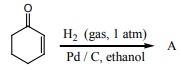# Chemistry - Online Test

Q1. For Freundlich isotherm a graph of log (x/m) is plotted against log p. The slope of the line and its y – axis intercept respectively corresponds to
Explaination / Solution:

x/m =k.p1/n

log(x/m )=logk+ 1/ n logp

y=c+mx

m = 1/n and c= logk

Q2. In which of the following , NH3 is not used?
Explaination / Solution:
No Explaination.

Q3. An alcohol (x) gives blue colour in Victormeyer’s test and 3.7g of X when treated with metallic sodium liberates 560 mL of hydrogen at 273 K and 1 atm pressure what will be the possible structure of X?
Explaination / Solution:

2 R - OH + 2Na 2 RONa + H22 moles of alcohol gives 1 mole of H2 which occupies 22.4L at 273K and 1 atm

number of moles of alcohol = ( 2 moles of R - OH / 22.4 L of H2 ) × 560 mL

= 0.05 moles

no. of moles = mass / molar mass

molar mass = 3.7/0.05 = 74 g mol1

General formula for R - OH Cn H2n+1 - OH

n(12) + (2n+1) (1) + 16+1=74

14n = 74 – 18

14n = 56

n = 56/14 = 4

The 2 alcohol which contains 4 carbon is CH2 CH(OH)CH2CH3

Q4. Sc( Z=21) is a transition element but Zinc (z=30) is not because
Explaination / Solution:
No Explaination.

Q5. The correct structure of the product ‘A’ formed in the reactionis
Explaination / Solution:
No Explaination.

Q6. The sum of primary valance and secondary valance of the metal M in the complex [ M ( en)2 ( Ox)] Cl is L
Explaination / Solution:

In the complex [M (en)2 (Ox)] Cl For the central metal ion M3+

The primary valance is = +3

The secondary valance = 6

sum of primary valance and secondary valance = 3+6 = 9

Q7. Which of the following reagent can be used to convert nitrobenzene to aniline
Explaination / Solution:
No Explaination.

Q8. Graphite and diamond are
Explaination / Solution:
No Explaination.

Q9. Which one of the following rotates the plane polarized light towards left?
Explaination / Solution:
No Explaination.

Q10. For a first order reaction A → B the rate constant is x min1 . If the initial concentration of A is 0.01M , the concentration of A after one hour is given by the expression.
Explaination / Solution:In this case

k = x min−1 and [A0] = 0.01M = 1 × 10−2M

t = 1 hour = 60 min

[A]= 1 × 10−2 ( e−60x)